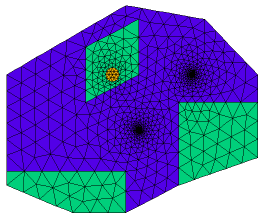﻿ Sample Problems > Applications > Groundwater > water

water

Navigation:  Sample Problems > Applications > Groundwater >

water{  WATER.PDE

This problem shows the flow of water to two wells, through soil regions of

differing porosity.  It also displays the ability of FlexPDE to grid features

of widely varying size.

}

title 'Groundwater flow to two wells'

definitions

k                   { no value is required, as long as it appears later }

s = 0               { no volumetric source }

k1 = 0.1             { high porosity value }

k2 = 1.0e-7         { low  porosity value }

sx1 = 0.7   sy1 = 0.4{ well 1 location }

sx2 = 0.5   sy2 = 0.2{ well 2 location }

srad = 0.001         { well radius = one thousandth of domain size }

w = 0.05             { a zoom window size }

px = 0.4    py = 0.4 { percolation pond center }

pr = 0.025           { percolation pond radius }

ps = 1e-4           { percolation rate }

variables

h

equations

h : div(k*grad(h)) + s = 0

boundaries

region 1 { The domain boundary, held at constant pressure head }

k=k1

start(0,0)

value(h)=0 line to (0.25,-0.1)

to (0.45,-0.1)

to (0.65,0)

to (0.95,0.1)

to (0.95,0.4)

to (0.75,0.6)

to (0.45,0.65)

to (0,0.4)

to close

{ Two wells, held at constant draw-down depths }

value(h) = -1       arc(center=sx1,sy1) angle=-360

value(h) = -2       arc(center=sx2,sy2) angle=-360

 region 2 { Some regions of low porosity }    k=k2   start(0,0) line to (0.25,-0.1)                   to (0.45,-0.1)                   to (0.45,0.05)                   to (0,0.05)                   to close   start(0.95,0.1) line to (0.95,0.3)                   to (0.65,0.3)                   to (0.65,0)                   to close   start(0.3,0.3) line to (0.5,0.4)                   to (0.5,0.6)                   to (0.3,0.5)                   to close region 3 { A percolation pond }    k = k2    s = ps { percolation rate }   start (px,py-pr) arc(center=px,py) angle=360monitors

contour(h)

plots

grid(x,y)

grid(x,y) zoom(sx1-w/2,sy1-w/2,w,w)

grid(x,y) zoom(sx2-w/2,sy2-w/2,w,w)